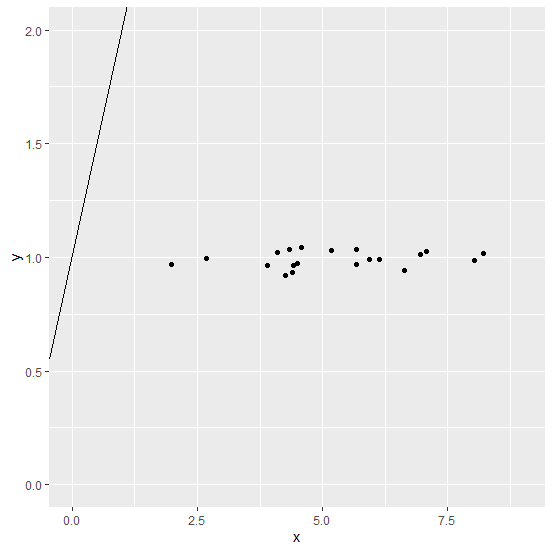# How to create scatterplot with intercept equals to 1 using ggplot2 in R?

To create a scatterplot with intercept equals to 1 using ggplot2, we can use geom_abline function but we need to pass the appropriate limits for the x axis and y axis values. For example, if we have two columns x and y in a data frame df and both have ranges starting from 0 to 5 then the scatterplot with intercept equals to 1 can be created as −

ggplot(df,aes(x,y))+geom_point()+geom_abline(intercept=1)+lims(x=c(0,5),y=c(0,5))

## Example

Consider the below data frame −

Live Demo

x<-rnorm(20,5,1.12)
y<-rnorm(20,1,0.035)
df<-data.frame(x,y)
df

## Output

      x       y
1 2.687278 0.9948582
2 4.410082 0.9646977
3 1.985577 0.9691989
4 8.218845 1.0174078
5 5.942984 0.9926087
6 4.346328 1.0340842
7 5.674543 0.9684308
8 6.138125 0.9891907
9 4.392059 0.9342166
10 8.028985 0.9871115
11 5.184612 1.0310949
12 3.896205 0.9636961
13 4.500758 0.9725633
14 6.950320 1.0140455
15 6.640275 0.9414716
16 7.071293 1.0262039
17 5.675679 1.0348059
18 4.104087 1.0214097
19 4.254315 0.9187824
20 4.585728 1.0420679

Loading ggplot2 package and the scatterplot with regression line having intercept equals to 1 −

## Example

library(ggplot2)
ggplot(df,aes(x,y))+geom_point()+geom_abline(intercept=1)+lims(x=c(0,9),y=c(0,2))

## Output